# CHAPTER Energy Energy Transfer And General Energy Analysis

• Slides: 54CHAPTER Energy, Energy Transfer And General Energy Analysis 1. Introduction 2. Forms of Energy 3. Energy Transfer by Heat 4. Energy Transfer by Work 5. Mechanical Forms of Work 6. The First Law of Thermodynamics 7. Energy Conservation Efficiencies 8. Energy And Environment 에너지변환시스템연구실(ECOS) Energy Conversion System Lab. 2 Chung H. Jeon2 -1 Introduction l We will soon learn how to apply the first law of thermodynamics as the expression of the conservation of energy principle. l But, first we study the ways in which energy may be transported across the boundary of a general thermodynamic system. l For closed systems (fixed mass systems) energy can cross the boundaries of a closed system only in the form of heat or work. l For open systems or control volumes energy can cross the control surface in the form of heat, work, and energy transported by the mass streams crossing the control surface. l We now consider each of these modes of energy transport across the boundaries of the general thermodynamic system. 에너지변환시스템연구실(ECOS) Energy Conversion System Lab. 2 Chung H. Jeon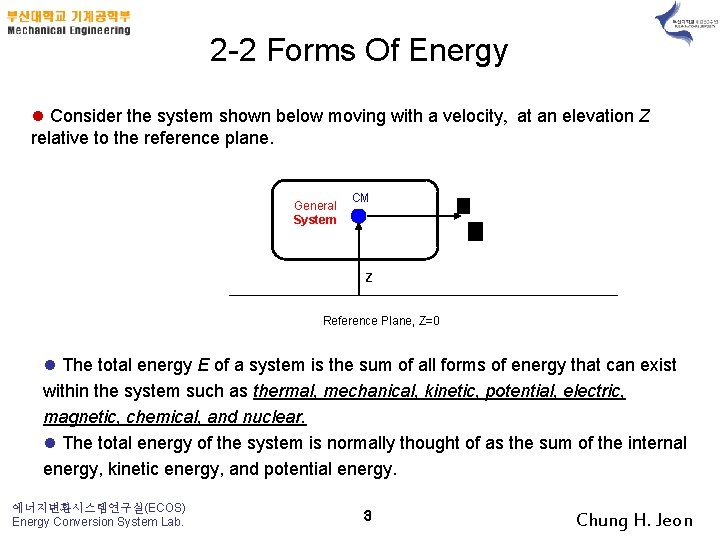2 -2 Forms Of Energy l Consider the system shown below moving with a velocity, at an elevation Z relative to the reference plane. General System CM Z Reference Plane, Z=0 l The total energy E of a system is the sum of all forms of energy that can exist within the system such as thermal, mechanical, kinetic, potential, electric, magnetic, chemical, and nuclear. l The total energy of the system is normally thought of as the sum of the internal energy, kinetic energy, and potential energy. 에너지변환시스템연구실(ECOS) Energy Conversion System Lab. 3 Chung H. Jeon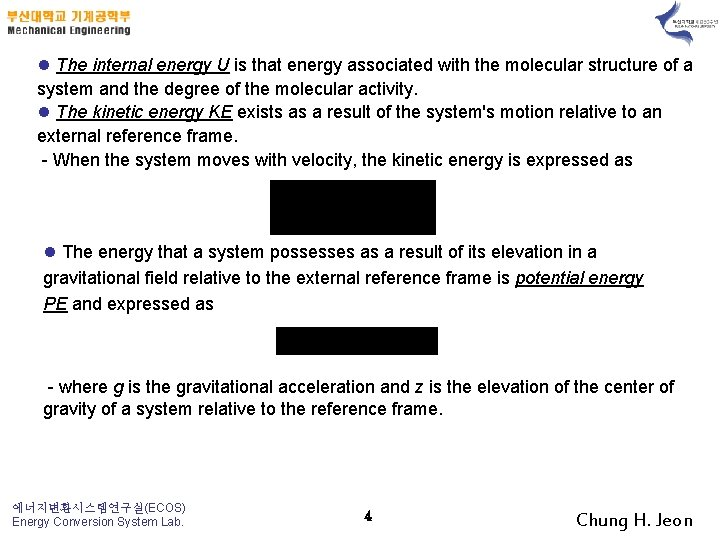l The internal energy U is that energy associated with the molecular structure of a system and the degree of the molecular activity. l The kinetic energy KE exists as a result of the system's motion relative to an external reference frame. - When the system moves with velocity, the kinetic energy is expressed as l The energy that a system possesses as a result of its elevation in a gravitational field relative to the external reference frame is potential energy PE and expressed as - where g is the gravitational acceleration and z is the elevation of the center of gravity of a system relative to the reference frame. 에너지변환시스템연구실(ECOS) Energy Conversion System Lab. 4 Chung H. Jeonl The total energy of the system is expressed as - where e = E/m is the specific energy, u = U/m is the specific internal energy l The change in stored energy of a system is given by l The change in the stored energy is identical to the change in internal energy for stationary systems. -> sensible and latent energy If KE = PE = 0, 에너지변환시스템연구실(ECOS) Energy Conversion System Lab. 5 Chung H. Jeon2 -3 Energy Transport by Heat l Recall that heat is energy in transition across the system boundary solely due to the temperature difference between the system and its surroundings. The net heat transferred to a system is defined as - Here, Qin and Qout are the magnitudes of the heat transfer values. l In most thermodynamics texts, the quantity Q is meant to be the net heat transferred to the system, Qnet. l Since heat transfer is process dependent, the differential of heat transfer Q is called inexact. We often think about the heat transfer per unit mass of the system, q. l Heat transfer has the units of energy measured in joules (we will use kilojoules, k. J) or the units of energy per unit mass, k. J/kg. 에너지변환시스템연구실(ECOS) Energy Conversion System Lab. 6 Chung H. Jeonl Since heat transfer is energy in transition across the system boundary due to a temperature difference l There are three modes of heat transfer at the boundary that depend on the temperature difference between the boundary surface and the surroundings. l These are conduction, convection, and radiation. l However, when solving problems in thermodynamics involving heat transfer to a system, the heat transfer is usually given or is calculated by applying the first law, or the conservation of energy, to the system. l An adiabatic process is one in which the system is perfectly insulated and the heat transfer is zero. 에너지변환시스템연구실(ECOS) Energy Conversion System Lab. 7 Chung H. Jeon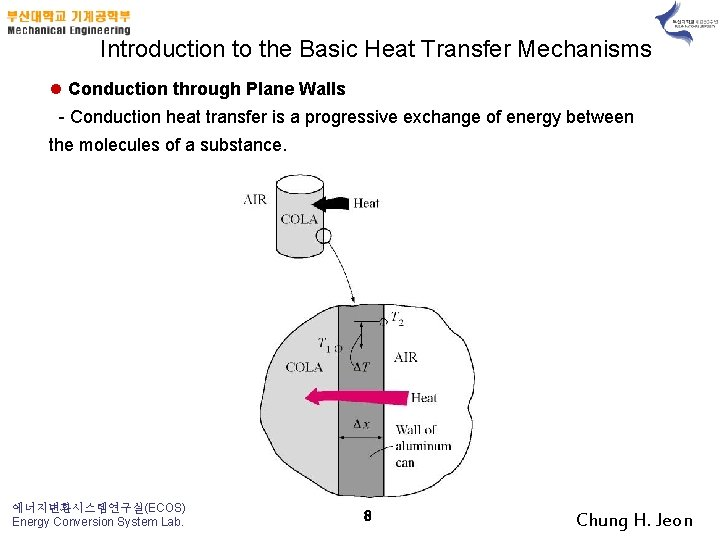Introduction to the Basic Heat Transfer Mechanisms l Conduction through Plane Walls - Conduction heat transfer is a progressive exchange of energy between the molecules of a substance. 에너지변환시스템연구실(ECOS) Energy Conversion System Lab. 8 Chung H. Jeon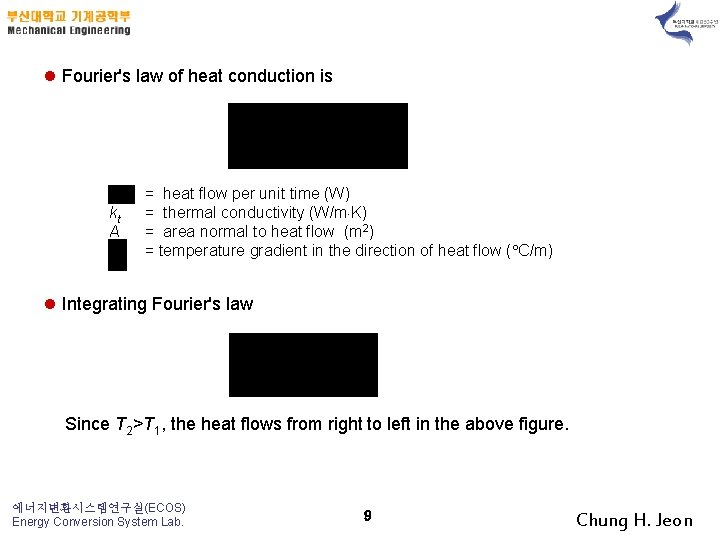l Fourier's law of heat conduction is kt A = heat flow per unit time (W) = thermal conductivity (W/m K) = area normal to heat flow (m 2) = temperature gradient in the direction of heat flow ( C/m) l Integrating Fourier's law Since T 2>T 1, the heat flows from right to left in the above figure. 에너지변환시스템연구실(ECOS) Energy Conversion System Lab. 9 Chung H. Jeon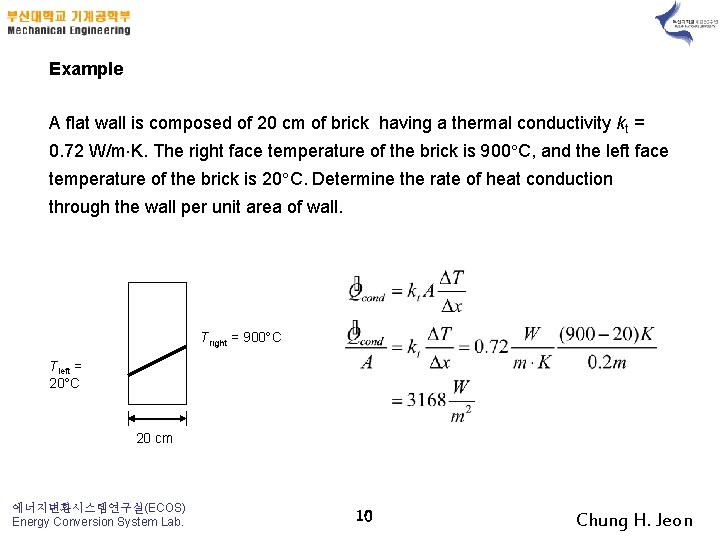Example A flat wall is composed of 20 cm of brick having a thermal conductivity kt = 0. 72 W/m K. The right face temperature of the brick is 900 C, and the left face temperature of the brick is 20 C. Determine the rate of heat conduction through the wall per unit area of wall. Tright = 900 C Tleft = 20 C 20 cm 에너지변환시스템연구실(ECOS) Energy Conversion System Lab. 10 Chung H. Jeon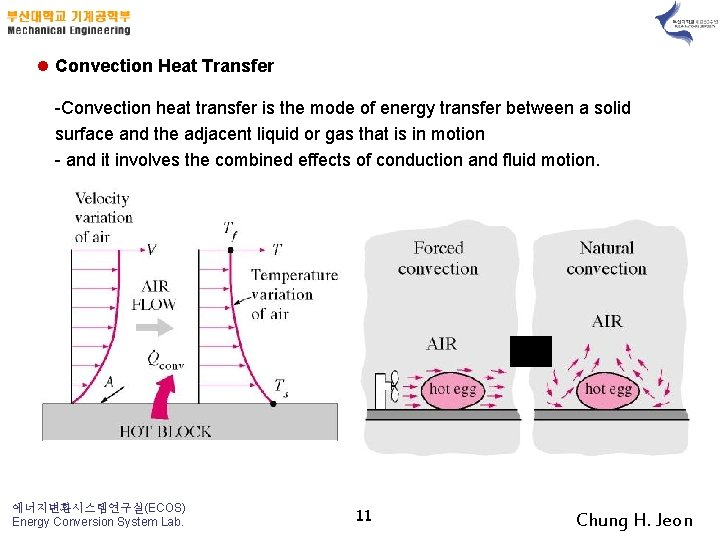l Convection Heat Transfer -Convection heat transfer is the mode of energy transfer between a solid surface and the adjacent liquid or gas that is in motion - and it involves the combined effects of conduction and fluid motion. 에너지변환시스템연구실(ECOS) Energy Conversion System Lab. 11 Chung H. Jeonl The rate of heat transfer by convection : Newton's law of cooling A h Ts Tf = heat transfer rate (W) = heat transfer area (m 2) = convective heat transfer coefficient (W/m 2 K) = surface temperature (K) = bulk fluid temperature away from the surface (K) l The convective heat transfer coefficient is an experimentally determined parameter that depends upon the surface geometry, the nature of the fluid motion, the properties of the fluid, and the bulk fluid velocity. l Ranges of the convective heat transfer coefficient are given below. h free convection of gases free convection of liquids forced convection of gases forced convection of liquids convection in boiling and condensation 에너지변환시스템연구실(ECOS) Energy Conversion System Lab. 12 W/m 2 K 2 -25 50 -100 25 -250 50 -20, 000 2500 -100, 000 Chung H. Jeon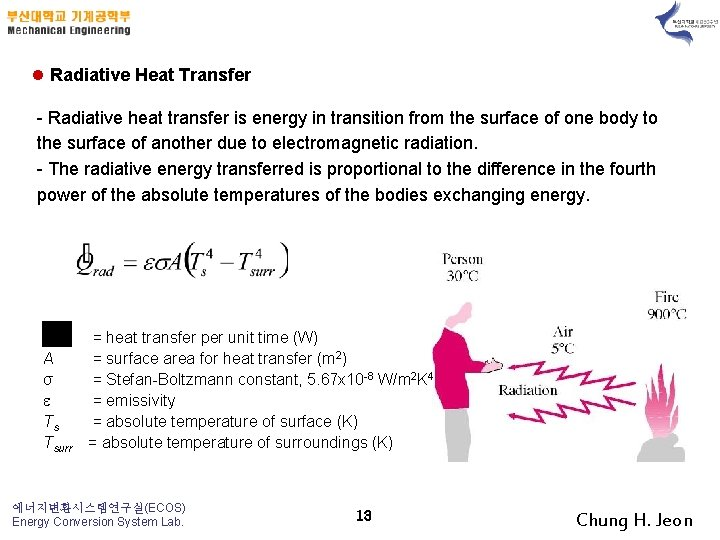l Radiative Heat Transfer - Radiative heat transfer is energy in transition from the surface of one body to the surface of another due to electromagnetic radiation. - The radiative energy transferred is proportional to the difference in the fourth power of the absolute temperatures of the bodies exchanging energy. A σ Ts Tsurr = heat transfer per unit time (W) = surface area for heat transfer (m 2) = Stefan-Boltzmann constant, 5. 67 x 10 -8 W/m 2 K 4 = emissivity = absolute temperature of surface (K) = absolute temperature of surroundings (K) 에너지변환시스템연구실(ECOS) Energy Conversion System Lab. 13 Chung H. JeonExample A vehicle is to be parked overnight in the open away from large surrounding objects. It is desired to know if dew or frost may form on the vehicle top. Assume the following: • Convection coefficient h from ambient air to vehicle top is 6. 0 W/m 2 C. • Equivalent sky temperature is -18 C. • Emissivity of vehicle top is 0. 84. • Negligible conduction from inside vehicle to top of vehicle. Determine the temperature of the vehicle top when the air temperature is 5 o. C. State which formation (dew or frost) occurs. 에너지변환시스템연구실(ECOS) Energy Conversion System Lab. 14 Chung H. JeonUnder steady-state conditions, the energy convected to the vehicle top is equal to the energy radiated to the sky. The energy convected from the ambient air to the vehicle top is The energy radiated from the top to the night sky is Setting these two heat transfers equal gives 에너지변환시스템연구실(ECOS) Energy Conversion System Lab. 15 Chung H. JeonWrite the equation for Ttop in C (T K = TC + 273) Using the EES software package Since Ttop is below the triple point of water, 0. 01 C, the water vapor in the air will form frost on the car top (see Chapter 14). 에너지변환시스템연구실(ECOS) Energy Conversion System Lab. 16 Chung H. Jeon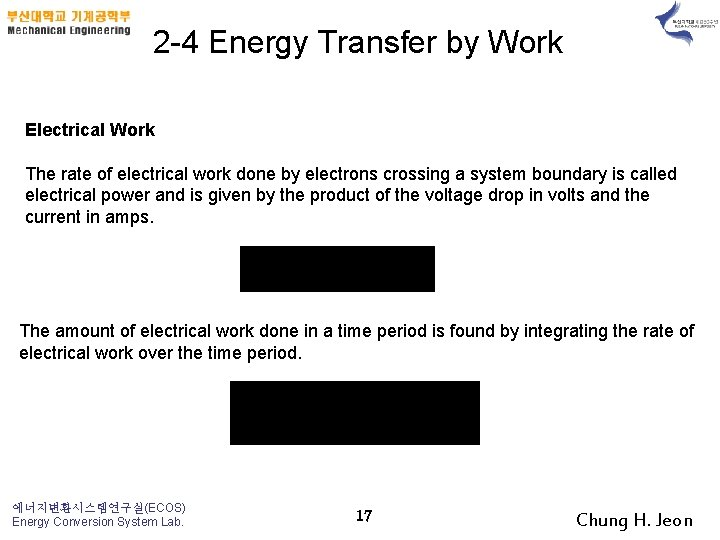2 -4 Energy Transfer by Work Electrical Work The rate of electrical work done by electrons crossing a system boundary is called electrical power and is given by the product of the voltage drop in volts and the current in amps. The amount of electrical work done in a time period is found by integrating the rate of electrical work over the time period. 에너지변환시스템연구실(ECOS) Energy Conversion System Lab. 17 Chung H. Jeon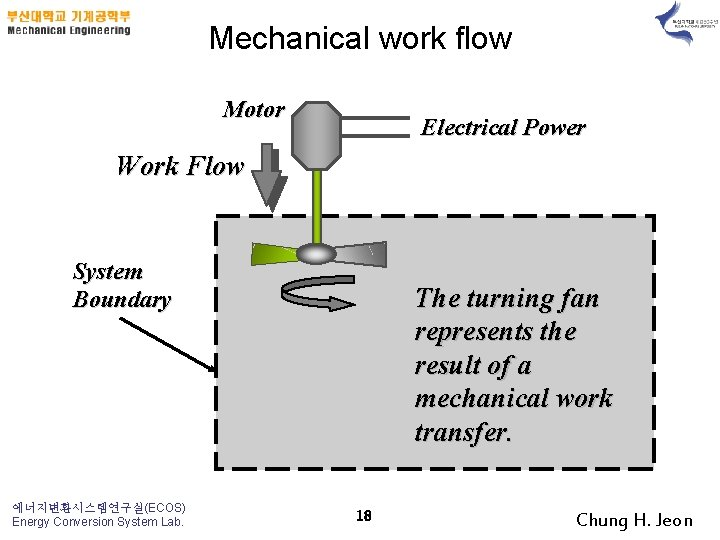Mechanical work flow Motor Electrical Power Work Flow System Boundary 에너지변환시스템연구실(ECOS) Energy Conversion System Lab. The turning fan represents the result of a mechanical work transfer. 18 Chung H. Jeon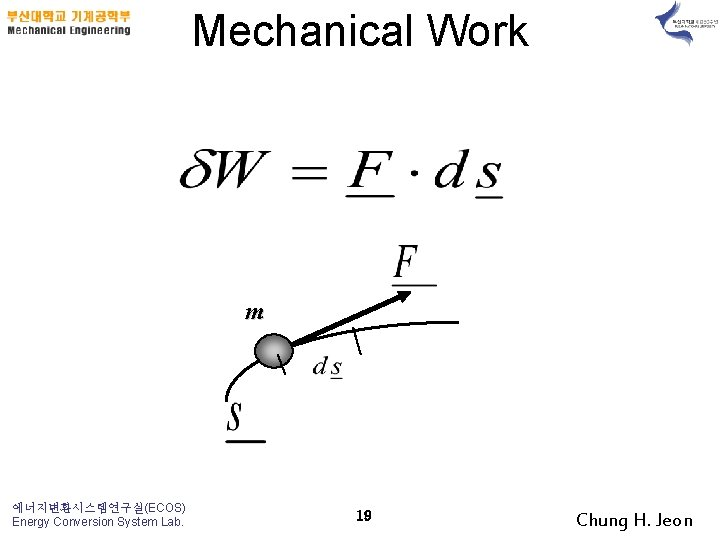Mechanical Work m 에너지변환시스템연구실(ECOS) Energy Conversion System Lab. 19 Chung H. Jeon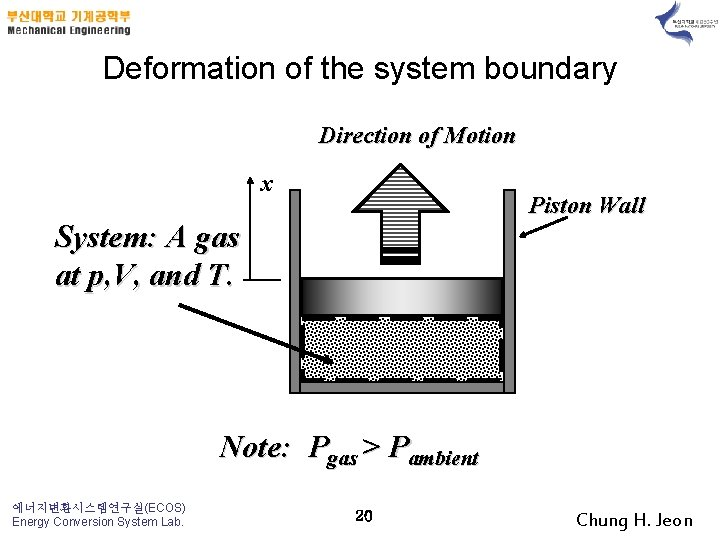Deformation of the system boundary Direction of Motion x Piston Wall System: A gas at p, V, and T. Note: Pgas > Pambient 에너지변환시스템연구실(ECOS) Energy Conversion System Lab. 20 Chung H. JeonWork transfer at a boundary Surroundings System W>0 W< 0 System Boundary 에너지변환시스템연구실(ECOS) Energy Conversion System Lab. 21 Chung H. Jeon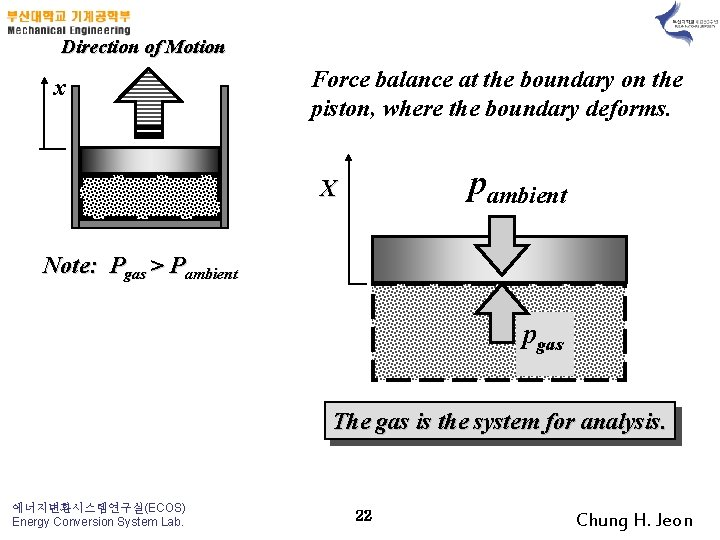Direction of Motion x Force balance at the boundary on the piston, where the boundary deforms. pambient X Note: Pgas > Pambient pgas The gas is the system for analysis. 에너지변환시스템연구실(ECOS) Energy Conversion System Lab. 22 Chung H. JeonX pambient pgas > pambient Component of work due to expansion of the gas 에너지변환시스템연구실(ECOS) Energy Conversion System Lab. 23 Work to raise the piston Chung H. Jeon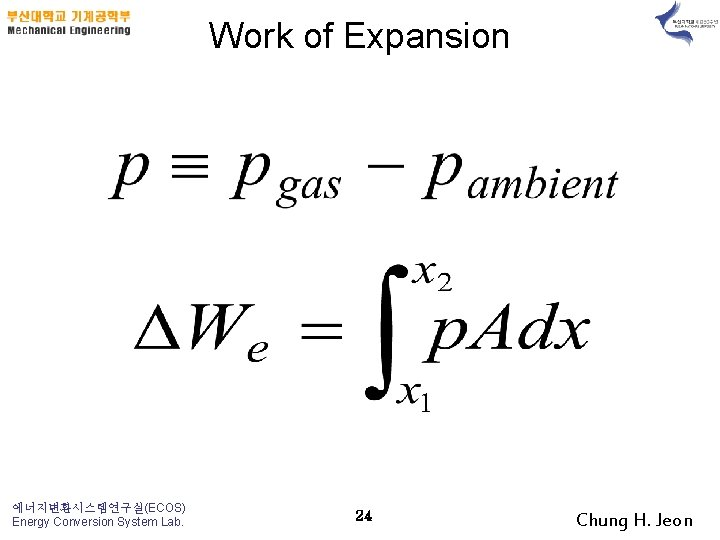Work of Expansion 에너지변환시스템연구실(ECOS) Energy Conversion System Lab. 24 Chung H. JeonWork of Expansion: p-d. V work 에너지변환시스템연구실(ECOS) Energy Conversion System Lab. 25 Chung H. JeonEvaluating a equilibrium expansion process p p 1 p 2 V 1 에너지변환시스템연구실(ECOS) Energy Conversion System Lab. V 2 26 V = Ax Chung H. Jeon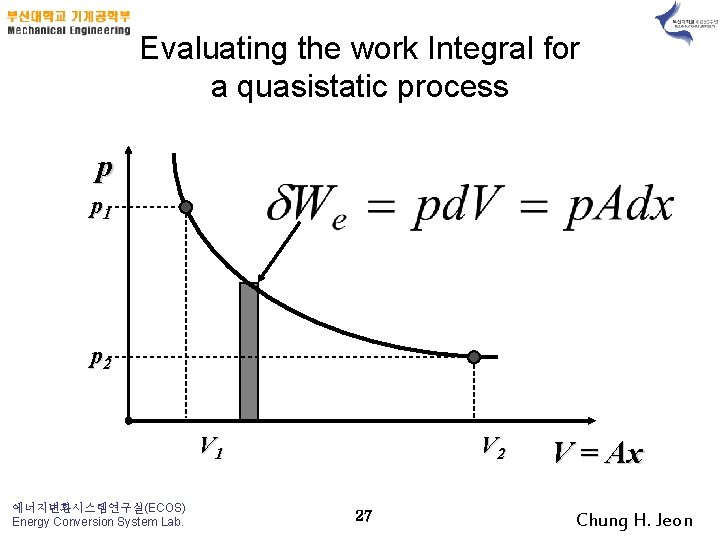Evaluating the work Integral for a quasistatic process p p 1 p 2 V 1 에너지변환시스템연구실(ECOS) Energy Conversion System Lab. V 2 27 V = Ax Chung H. Jeon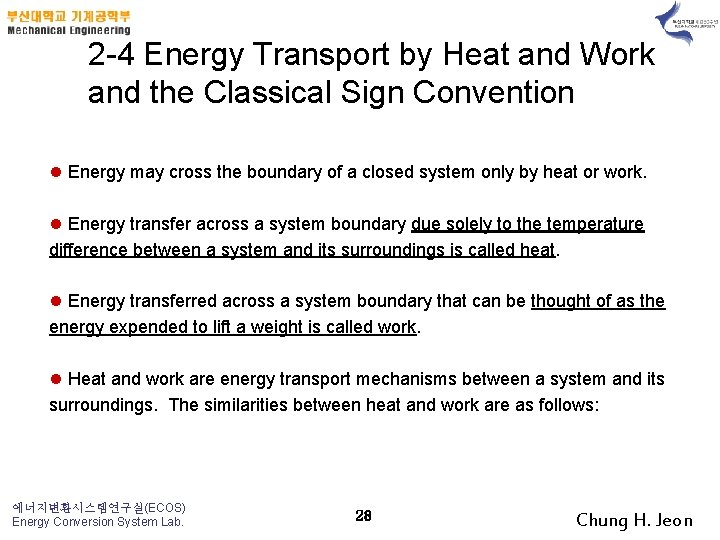2 -4 Energy Transport by Heat and Work and the Classical Sign Convention l Energy may cross the boundary of a closed system only by heat or work. l Energy transfer across a system boundary due solely to the temperature difference between a system and its surroundings is called heat. l Energy transferred across a system boundary that can be thought of as the energy expended to lift a weight is called work. l Heat and work are energy transport mechanisms between a system and its surroundings. The similarities between heat and work are as follows: 에너지변환시스템연구실(ECOS) Energy Conversion System Lab. 28 Chung H. Jeon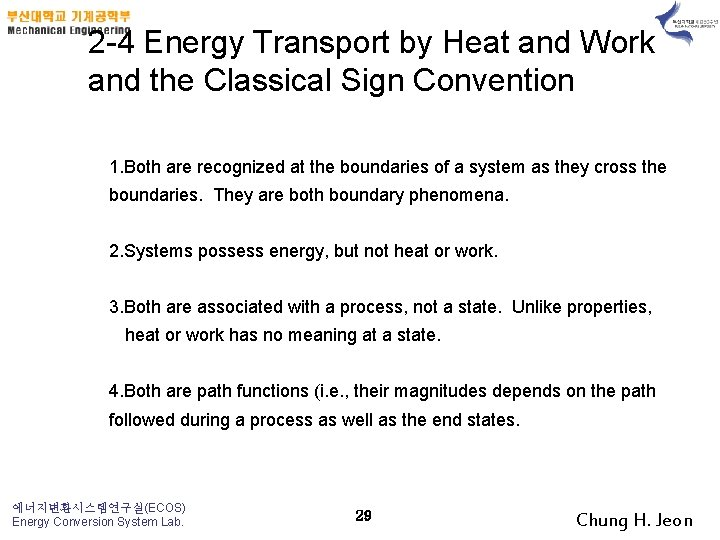2 -4 Energy Transport by Heat and Work and the Classical Sign Convention 1. Both are recognized at the boundaries of a system as they cross the boundaries. They are both boundary phenomena. 2. Systems possess energy, but not heat or work. 3. Both are associated with a process, not a state. Unlike properties, heat or work has no meaning at a state. 4. Both are path functions (i. e. , their magnitudes depends on the path followed during a process as well as the end states. 에너지변환시스템연구실(ECOS) Energy Conversion System Lab. 29 Chung H. Jeonl Since heat and work are path dependent functions, they have inexact differentials designated by the symbol . l The differentials of heat and work are expressed as Q and W. l The integral of the differentials of heat and work over the process path gives the amount of heat or work transfer that occurred at the system boundary during a process. l. The total heat transfer or work is obtained by following the process path and adding the differential amounts of heat ( Q) or work ( W) along the way. l The integrals of Q and W are not Q 2 – Q 1 and W 2 – W 1, respectively, which are meaningless since both heat and work are not properties and systems do not possess heat or work at a state. 에너지변환시스템연구실(ECOS) Energy Conversion System Lab. 30 Chung H. Jeon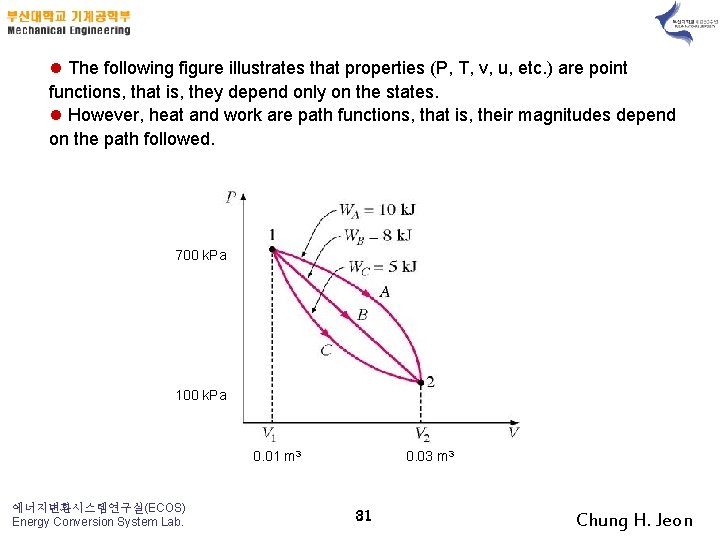l The following figure illustrates that properties (P, T, v, u, etc. ) are point functions, that is, they depend only on the states. l However, heat and work are path functions, that is, their magnitudes depend on the path followed. 700 k. Pa 100 k. Pa 0. 01 m 3 에너지변환시스템연구실(ECOS) Energy Conversion System Lab. 0. 03 m 3 31 Chung H. Jeonl A sign convention is required for heat and work energy transfers, and the classical thermodynamic sign convention is selected for these notes. l Heat transfer to a system and work done by a system are positive l Heat transfer from a system and work a system are negative. l The system shown below has heat supplied to it and work done by it. System Boundary 에너지변환시스템연구실(ECOS) Energy Conversion System Lab. 32 Chung H. Jeon2 -5 Mechanical Forms of Work l Work is energy expended by a force acting through a distance. l Thermodynamic work is defined as energy in transition across the system boundary and is done by a system if the sole effect external to the boundaries could have been the raising of a weight. - here is the angle between the force vector and the displacement vector. 에너지변환시스템연구실(ECOS) Energy Conversion System Lab. 33 Chung H. Jeon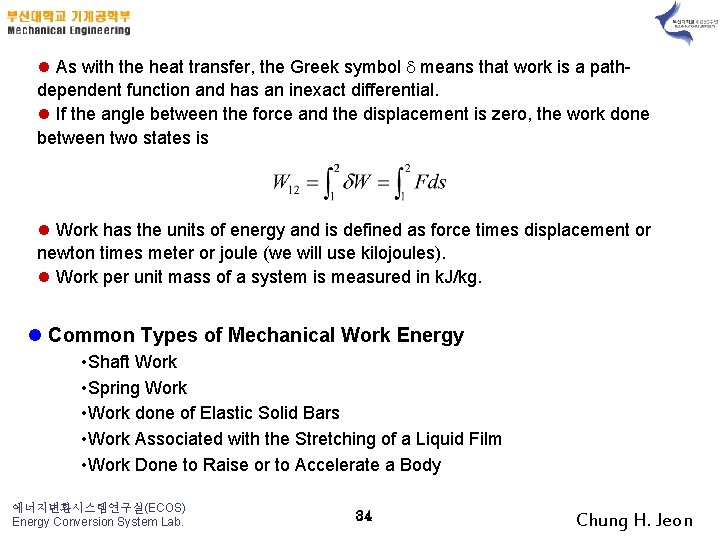l As with the heat transfer, the Greek symbol means that work is a pathdependent function and has an inexact differential. l If the angle between the force and the displacement is zero, the work done between two states is l Work has the units of energy and is defined as force times displacement or newton times meter or joule (we will use kilojoules). l Work per unit mass of a system is measured in k. J/kg. l Common Types of Mechanical Work Energy • Shaft Work • Spring Work • Work done of Elastic Solid Bars • Work Associated with the Stretching of a Liquid Film • Work Done to Raise or to Accelerate a Body 에너지변환시스템연구실(ECOS) Energy Conversion System Lab. 34 Chung H. Jeonl Net Work Done By A System l The net work done by a system may be in two forms other work and boundary work. l First, work may cross a system boundary in the form of a rotating shaft work, electrical work or other the work forms listed above. - We will call these work forms “other” work, that is, work not associated with a moving boundary. - In thermodynamics electrical energy is normally considered to be work energy rather than heat energy - however, the placement of the system boundary dictates whether to include electrical energy as work or heat. l Second, the system may do work on its surroundings because of moving boundaries due to expansion or compression processes that a fluid may experience in a piston-cylinder device. 에너지변환시스템연구실(ECOS) Energy Conversion System Lab. 35 Chung H. Jeon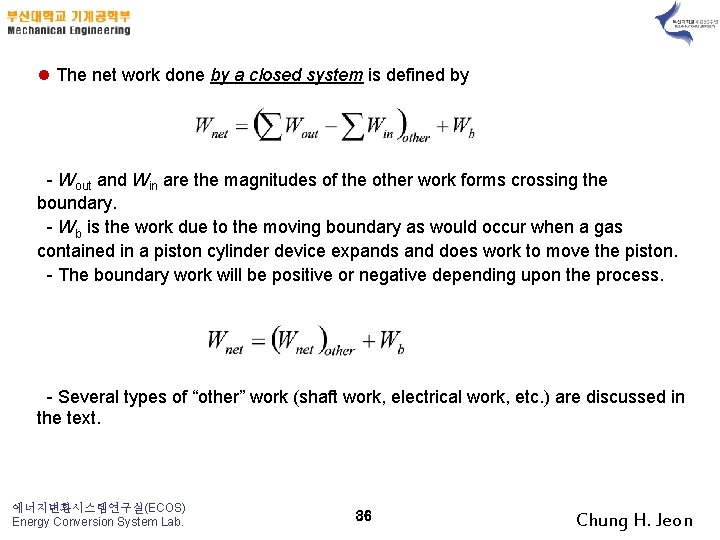l The net work done by a closed system is defined by - Wout and Win are the magnitudes of the other work forms crossing the boundary. - Wb is the work due to the moving boundary as would occur when a gas contained in a piston cylinder device expands and does work to move the piston. - The boundary work will be positive or negative depending upon the process. - Several types of “other” work (shaft work, electrical work, etc. ) are discussed in the text. 에너지변환시스템연구실(ECOS) Energy Conversion System Lab. 36 Chung H. JeonExample A fluid contained in a piston-cylinder device receives 500 k. J of electrical work as the gas expands against the piston and does 600 k. J of boundary work on the piston. What is the net work done by the fluid? Wb=600 k. J Wele =500 k. J 에너지변환시스템연구실(ECOS) Energy Conversion System Lab. 37 Chung H. Jeon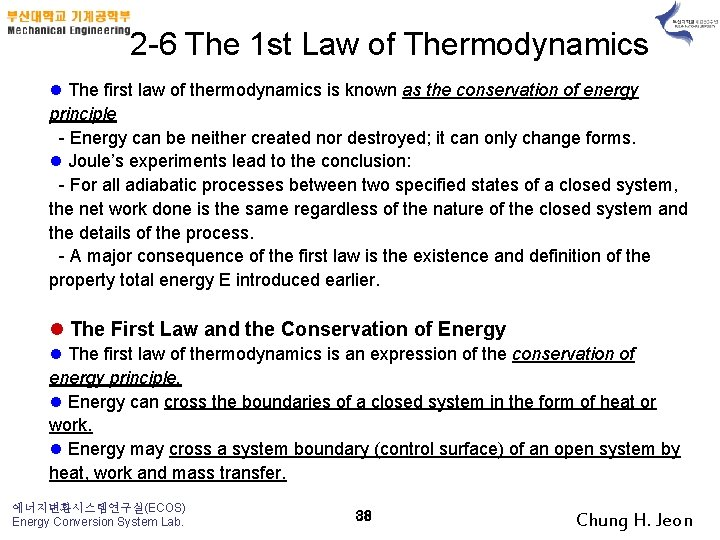2 -6 The 1 st Law of Thermodynamics l The first law of thermodynamics is known as the conservation of energy principle - Energy can be neither created nor destroyed; it can only change forms. l Joule’s experiments lead to the conclusion: - For all adiabatic processes between two specified states of a closed system, the net work done is the same regardless of the nature of the closed system and the details of the process. - A major consequence of the first law is the existence and definition of the property total energy E introduced earlier. l The First Law and the Conservation of Energy l The first law of thermodynamics is an expression of the conservation of energy principle. l Energy can cross the boundaries of a closed system in the form of heat or work. l Energy may cross a system boundary (control surface) of an open system by heat, work and mass transfer. 에너지변환시스템연구실(ECOS) Energy Conversion System Lab. 38 Chung H. Jeon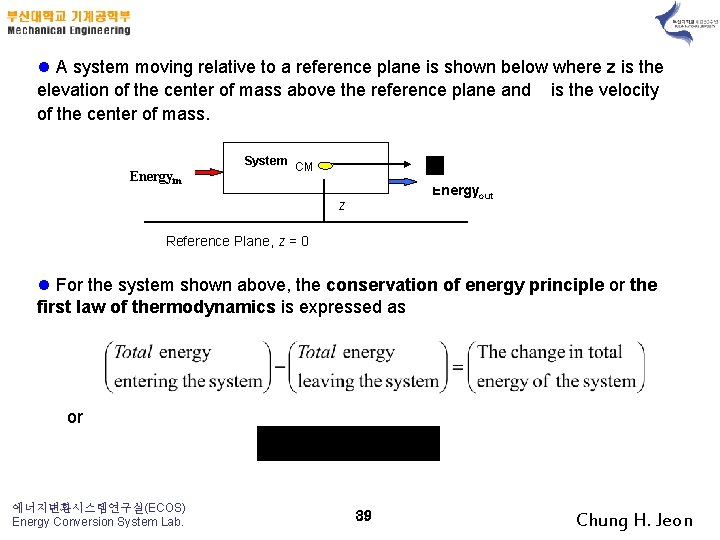l A system moving relative to a reference plane is shown below where z is the elevation of the center of mass above the reference plane and is the velocity of the center of mass. System Energyin CM Energyout z Reference Plane, z = 0 l For the system shown above, the conservation of energy principle or the first law of thermodynamics is expressed as or 에너지변환시스템연구실(ECOS) Energy Conversion System Lab. 39 Chung H. Jeon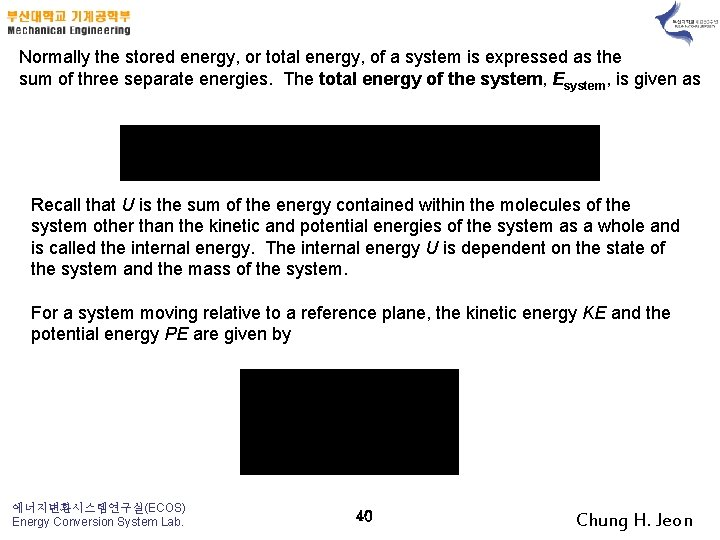Normally the stored energy, or total energy, of a system is expressed as the sum of three separate energies. The total energy of the system, Esystem, is given as Recall that U is the sum of the energy contained within the molecules of the system other than the kinetic and potential energies of the system as a whole and is called the internal energy. The internal energy U is dependent on the state of the system and the mass of the system. For a system moving relative to a reference plane, the kinetic energy KE and the potential energy PE are given by 에너지변환시스템연구실(ECOS) Energy Conversion System Lab. 40 Chung H. Jeon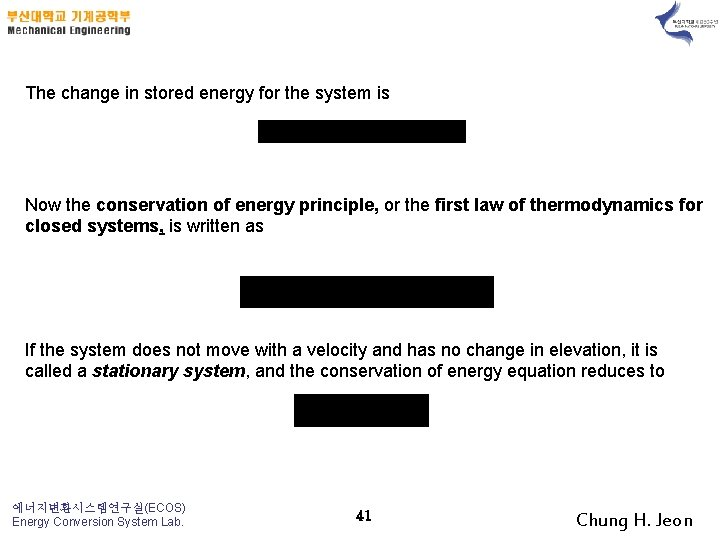The change in stored energy for the system is Now the conservation of energy principle, or the first law of thermodynamics for closed systems, is written as If the system does not move with a velocity and has no change in elevation, it is called a stationary system, and the conservation of energy equation reduces to 에너지변환시스템연구실(ECOS) Energy Conversion System Lab. 41 Chung H. Jeon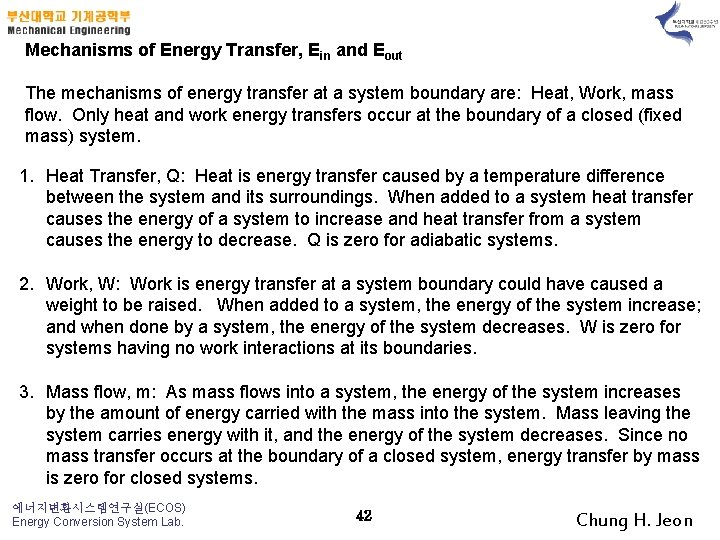Mechanisms of Energy Transfer, Ein and Eout The mechanisms of energy transfer at a system boundary are: Heat, Work, mass flow. Only heat and work energy transfers occur at the boundary of a closed (fixed mass) system. 1. Heat Transfer, Q: Heat is energy transfer caused by a temperature difference between the system and its surroundings. When added to a system heat transfer causes the energy of a system to increase and heat transfer from a system causes the energy to decrease. Q is zero for adiabatic systems. 2. Work, W: Work is energy transfer at a system boundary could have caused a weight to be raised. When added to a system, the energy of the system increase; and when done by a system, the energy of the system decreases. W is zero for systems having no work interactions at its boundaries. 3. Mass flow, m: As mass flows into a system, the energy of the system increases by the amount of energy carried with the mass into the system. Mass leaving the system carries energy with it, and the energy of the system decreases. Since no mass transfer occurs at the boundary of a closed system, energy transfer by mass is zero for closed systems. 에너지변환시스템연구실(ECOS) Energy Conversion System Lab. 42 Chung H. JeonThe energy balance for a general system is Expressed more compactly, the energy balance is or on a rate form, as 에너지변환시스템연구실(ECOS) Energy Conversion System Lab. 43 Chung H. Jeon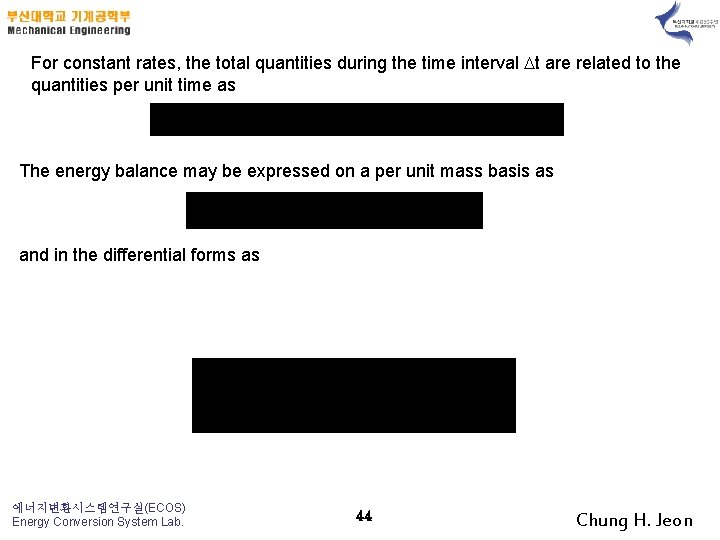For constant rates, the total quantities during the time interval t are related to the quantities per unit time as The energy balance may be expressed on a per unit mass basis as and in the differential forms as 에너지변환시스템연구실(ECOS) Energy Conversion System Lab. 44 Chung H. Jeon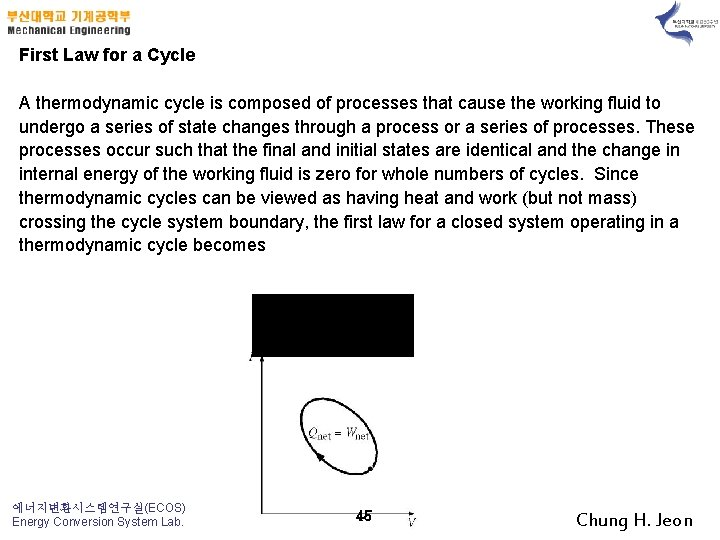First Law for a Cycle A thermodynamic cycle is composed of processes that cause the working fluid to undergo a series of state changes through a process or a series of processes. These processes occur such that the final and initial states are identical and the change in internal energy of the working fluid is zero for whole numbers of cycles. Since thermodynamic cycles can be viewed as having heat and work (but not mass) crossing the cycle system boundary, the first law for a closed system operating in a thermodynamic cycle becomes 에너지변환시스템연구실(ECOS) Energy Conversion System Lab. 45 Chung H. Jeon2 -7 Energy Conversion Efficiencies A measure of performance for a device is its efficiency and is often given the symbol . Efficiencies are expressed as follows: How will you measure your efficiency in this thermodynamics course? Efficiency as the Measure of Performance of a Thermodynamic cycle A system has completed a thermodynamic cycle when the working fluid undergoes a series of processes and then returns to its original state, so that the properties of the system at the end of the cycle are the same as at its beginning. Thus, for whole numbers of cycles 에너지변환시스템연구실(ECOS) Energy Conversion System Lab. 46 Chung H. Jeon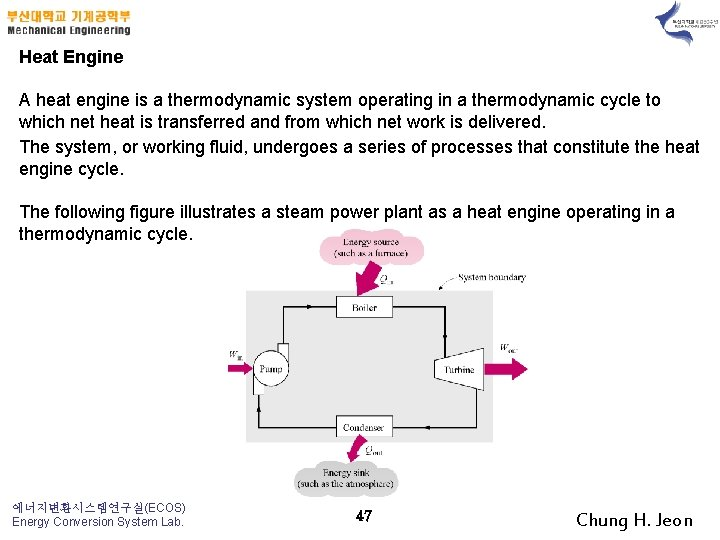Heat Engine A heat engine is a thermodynamic system operating in a thermodynamic cycle to which net heat is transferred and from which net work is delivered. The system, or working fluid, undergoes a series of processes that constitute the heat engine cycle. The following figure illustrates a steam power plant as a heat engine operating in a thermodynamic cycle. 에너지변환시스템연구실(ECOS) Energy Conversion System Lab. 47 Chung H. JeonThermal Efficiency, th The thermal efficiency is the index of performance of a work-producing device or a heat engine and is defined by the ratio of the net work output (the desired result) to the heat input (the cost or required input to obtain the desired result). For a heat engine the desired result is the net work done (Wout – Win) and the input is the heat supplied to make the cycle operate Qin. The thermal efficiency is always less than 1 or less than 100 percent. where Here, the use of the in and out subscripts means to use the magnitude (take the positive value) of either the work or heat transfer and let the minus sign in the net expression take care of the direction. 에너지변환시스템연구실(ECOS) Energy Conversion System Lab. 48 Chung H. Jeon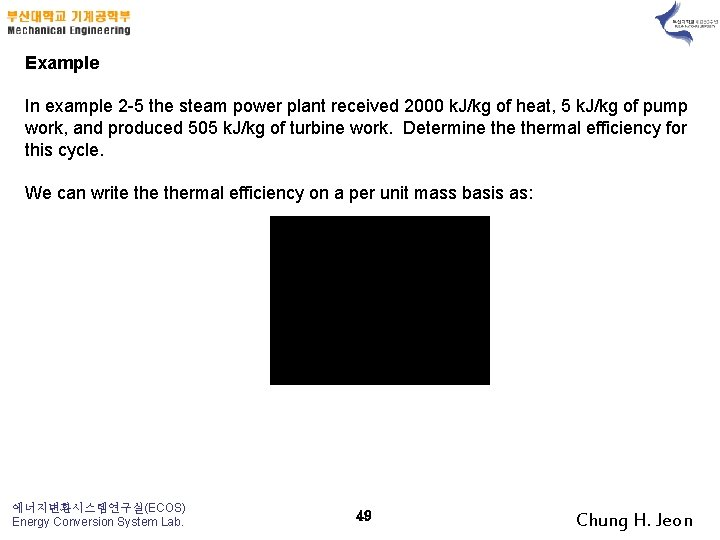Example In example 2 -5 the steam power plant received 2000 k. J/kg of heat, 5 k. J/kg of pump work, and produced 505 k. J/kg of turbine work. Determine thermal efficiency for this cycle. We can write thermal efficiency on a per unit mass basis as: 에너지변환시스템연구실(ECOS) Energy Conversion System Lab. 49 Chung H. JeonCombustion Efficiency Consider the combustion of a fuel-air mixture as shown below. Fuel Cn. Hm Combustion Chamber Air Reactants T R , PR Qout = HV CO 2 H 2 O N 2 Products PP, TP Fuels are usually composed of a compound or mixture containing carbon, C, and hydrogen, H 2. During a complete combustion process all of the carbon is converted to carbon dioxide and all of the hydrogen is converted to water. For stoichiometric combustion (theoretically correct amount of air is supplied for complete combustion) where both the reactants (fuel plus air) and the products (compounds formed during the combustion process) have the same temperatures, the heat transfer from the combustion process is called the heating value of the fuel. 에너지변환시스템연구실(ECOS) Energy Conversion System Lab. 50 Chung H. JeonThe lower heating value, LHV, is the heating value when water appears as a gas in the products. The lower heating value is often used as the measure of energy per kg of fuel supplied to the gas turbine engine because the exhaust gases have such a high temperature that the water formed is a vapor as it leaves the engine with other products of combustion. The higher heating value, HHV, is the heating value when water appears as a liquid in the products. The higher heating value is often used as the measure of energy per kg of fuel supplied to the steam power cycle because there are heat transfer processes within the cycle that absorb enough energy from the products of combustion that some of the water vapor formed during combustion will condense. Combustion efficiency is the ratio of the actual heat transfer from the combustion process to the heating value of the fuel. 에너지변환시스템연구실(ECOS) Energy Conversion System Lab. 51 Chung H. Jeon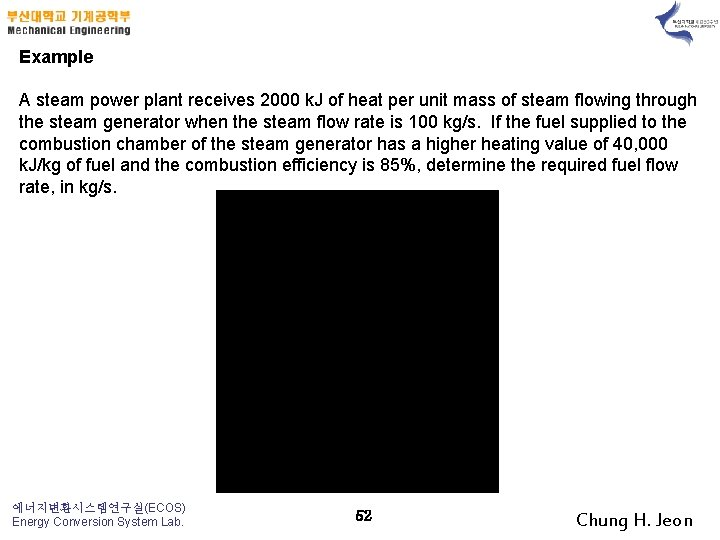Example A steam power plant receives 2000 k. J of heat per unit mass of steam flowing through the steam generator when the steam flow rate is 100 kg/s. If the fuel supplied to the combustion chamber of the steam generator has a higher heating value of 40, 000 k. J/kg of fuel and the combustion efficiency is 85%, determine the required fuel flow rate, in kg/s. 에너지변환시스템연구실(ECOS) Energy Conversion System Lab. 52 Chung H. JeonGenerator Efficiency: Power Plant Overall Efficiency: Motor Efficiency: 에너지변환시스템연구실(ECOS) Energy Conversion System Lab. 53 Chung H. Jeon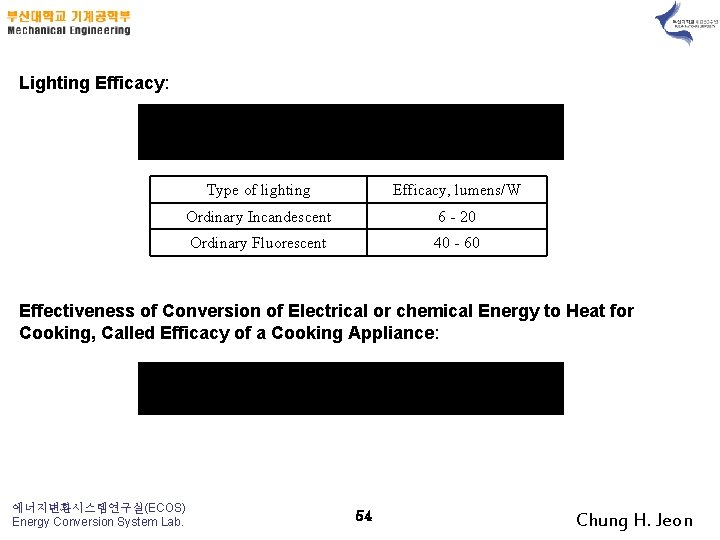Lighting Efficacy: Type of lighting Efficacy, lumens/W Ordinary Incandescent 6 - 20 Ordinary Fluorescent 40 - 60 Effectiveness of Conversion of Electrical or chemical Energy to Heat for Cooking, Called Efficacy of a Cooking Appliance: 에너지변환시스템연구실(ECOS) Energy Conversion System Lab. 54 Chung H. Jeon